The Crafoord prize is a prestigious prize administered by the Swedish academy of sciences. Not as prestigious as the Nobel, it is still a highly respectable prize that comes with a respectable sum of money.

This way, one of the recipients was Roy Kerr, known for his solution of rotating black holes.

Several people were invited to give talks, including Roy Kerr’s colleague David Wiltshire. Wiltshire began his talk by mentioning the role of a young John Moffat in inspiring Kerr to study the rotating solution, but he also acknowledged Moffat’s more recent work, in which I also played a role, his Scalar-Tensor-Vector (STVG) modified gravity theory, aka MOG.

All too often, MOG is ignored, dismissed or confused with other theories. It was very good to see a rare, notable exception from that rule.

This morning, Quora surprised me with this:Say what?

I have written a grand total of three Quora answers related to the Quran (or Koran, which is the spelling I prefer). Two of these were just quoting St. Augustine of Hippo, an early Christian saint who advised Christians not to confuse the Book of Genesis with science; the third was about a poll from a few years back that showed that in the United States, atheists/agnostics know more about religion than religious folk from any denomination.

As to string theory, I try to avoid the topic because I don’t know enough about it. Still, 15 of my answers on related topics (particle physics, cosmology) were apparently also categorized under the String Theory label.

But I fail to see how my contributions make me an expert on either Islam or String Theory.

Not for the first time, I am reading a paper that discusses the dark matter paradigm and its alternatives.

Except that it doesn’t. Discuss the alternatives, that is. It discusses the one alternative every schoolchild interested in the sciences knows about (and one that, incidentally, doesn’t really work) while ignoring the rest.

This one alternative is Mordehai Milgrom’s MOND, or MOdified Newtonian Dynamics, and its generalization, TeVeS (Tensor-Vector-Scalar theory) by the late Jacob Bekenstein.

Unfortunately, too many people think that MOND is the only game in town, or that even if it isn’t, it is somehow representative of its alternatives. But it is not.

In particular, I find it tremendously annoying when people confuse MOND with Moffat’s MOG (MOdified Gravity, also MOffat Gravity). Or when similarly, they confuse TeVeS with STVG (Scalar-tensor-Vector Gravity), which is the relativistic theory behind the MOG phenomenology.

So how do they differ?

MOND is a phenomenological postulate concerning a minimum acceleration. It modifies Newton’s second law: Instead of $$F = ma$$, we have $$F = m\mu(a/a_0)a$$, where $$\mu(x)$$ is a function that satisfies $$\mu(x)\to 1$$ for $$x\gg 1$$, and $$\mu(x)\to x$$ for $$x\ll 1$$. A good example would be $$\mu(x)=1/(1+1/x)$$. The magnitude of the MOND acceleration is $$a_0={\cal O}(10^{-10})~{\rm m}/{\rm s}$$.

The problem with MOND is that in this form, it violates even basic conservation laws. It is not a theory: it is just a phenomenological formula designed to explain the anomalous rotation curves of spiral galaxies.

MOND was made more respectable by Jacob Bekenstein, who constructed a relativistic field theory of gravity that approximately reproduces the MOND acceleration law in the non-relativistic limit. The theory incorporates a unit 4-vector field and a scalar field. It also has the characteristics of a bimetric theory, in that a “physical metric” is constructed from the true metric and the vector field, and this physical metric determines the behavior of ordinary matter.

In contrast, MOG is essentially a Yukawa theory of gravity in the weak field approximation, with two twists. The first twist is that in MOG, attractive gravity is stronger than Newton’s or Einstein’s; however, at a finite range, it is counteracted by a repulsive force, so the gravitational acceleration is in fact given by $$a = GM[1+\alpha-\alpha(1+\mu r)e^{-\mu r}]$$, where $$\alpha$$ determines the strength of attractive gravity ($$\alpha=0$$ means Newtonian gravity) and $$\mu$$ is the range of the vector force. (Typically, $$\alpha={\cal O}(1)$$, $$\mu^{-1}={\cal O}(10)~{\rm kpc}$$.) The second twist is that the strength of attractive gravity and the range of the repulsive force are both variable, i.e., dynamical (though possibly algebraically related) degrees of freedom. And unlike MOND, for which a relativistic theory was constructed after-the-fact, MOG is derived from a relativistic field theory. It, too, includes a vector field and one or two scalar fields, but the vector field is not a unit vector field, and there is no additional, “physical metric”.

In short, there is not even a superficial resemblance between the two theories. Moreover, unlike MOND, MOG has a reasonably good track record dealing with things other than galaxies: this includes globular clusters (for which MOND has to invoke the nebulous “external field effect”), cluster of galaxies (including the famous Bullet Cluster, seen by some as incontrovertible proof that dark matter exists) and cosmology (for which MOND requires something like 2 eV neutrinos to be able to fit the data.)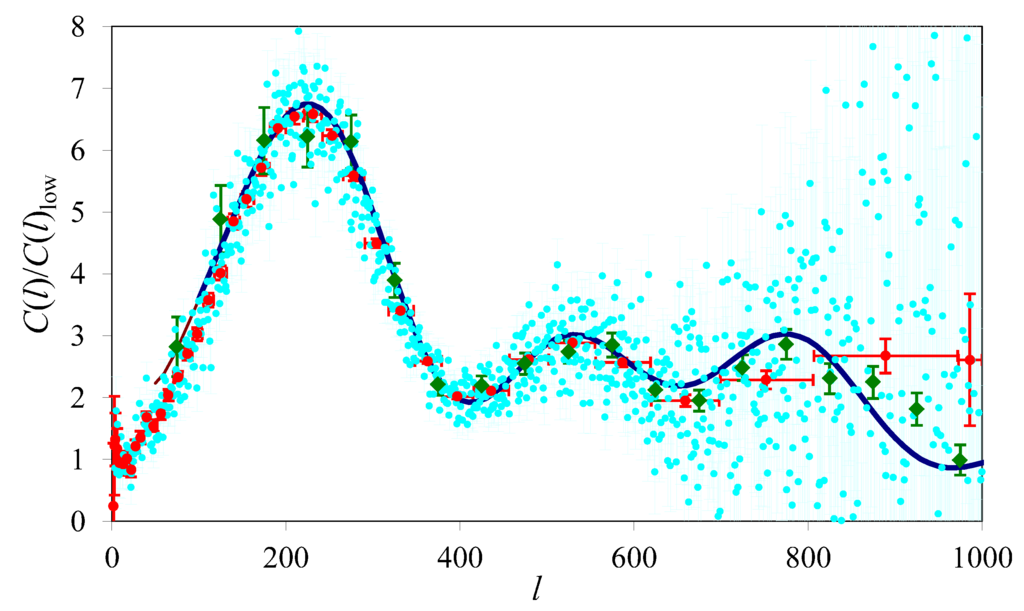MOG and the acoustic power spectrum. Calculated using $$\Omega_M=0.3$$, $$\Omega_b=0.035$$, $$H_0=71~{\rm km}/{\rm s}/{\rm Mpc}$$. Also shown are the raw Wilkinson Microwave Anisotropy Probe (WMAP) three-year data set (light blue), binned averages with horizontal and vertical error bars provided by the WMAP project (red) and data from the Boomerang experiment (green). From arXiv:1104.2957.

There are many issues with MOG, to be sure. Personally, I have never been satisfied with the way we treated the scalar field so far, and I’d really like to be able to derive a proper linearized version of the theory in which the scalar field, too, is accommodated as a first-class citizen. How MOG stands up to scrutiny in light of precision solar system data at the PPN level is also an open question.

But to see MOG completely ignored in the literature, and see MOND used essentially as a straw man supposedly representing all attempts at creating a modified gravity alternative to dark matter… that is very disheartening.

This is an eerie anniversary.

Thirty years ago today, reactor 4 of the Chernobyl nuclear power plant blew to smithereens.

It’s really hard to assign blame.

Was it the designers who came up with a reactor design that was fundamentally unstable at low power?

Was it the bureaucrats who, in the secretive Soviet polie state, made it hard if not impossible for operators at one facility to learn from incidents elsewhere?

Was it the engineers at Chernobyl who, concerned about the consequences of a total loss of power at the station, tried to test a procedure that would have kept control systems and the all-important coolant pumps running using waste heat during an emergency shutdown, while the Diesel generators kicked in?

Was it the Kiev electricity network operator who asked Chernobyl to keep reactor 4 online for a little longer, thus pushing the planned test into the late night?

Was it the control room operator who ultimately pushed the button that initiated an emergency shutdown?

And the list continues. Many of the people we could blame didn’t stick around long enough: they died, after participating in often heroic efforts to avert an even greater disaster, and receiving lethal doses of radiation.Some lived. This photo shows Arkady Uskov, who suffered severe radiation burns 30 years ago as he helped save colleagues. He, along with a few other people, recently revisited the control room of reactor 4, and were photographed there by Radio Free Europe. (Sadly, the photos are badly mislabeled by someone who didn’t know that “Arcadia Uskova” would be the name of a female; or, in this case, the genitive case of the male name Arkady Uskov. Thus I also cannot tell if “Oleksandr Cheranov”, whose name I cannot find anywhere else in the literature of Chernobyl, was a real person or just another RFE misprint.)

Surprisingly, the control room, which looks like a set of props from a Cold War era science fiction movie, is still partially alive. The lit panels, I suspect, must be either part of the monitoring effort or communications equipment.

It must have been an uncanny feeling for these aging engineers to be back at the scene, 30 years later, contemplating what took place that night.

Incidentally, nuclear power remains by far the safest in the world. Per unit of energy produced, it is dozens of times safer than hydroelectricity; a hundred times safer than natural gas; and a whopping four thousand times safer than coal. And yes, this includes the additional approximately 4,000 premature deaths (UN estimate) as a result of Chernobyl’s fallout. Nor was Chernobyl the deadliest accident related to power generation; that title belongs to China’s Banqiao Dam, the failure of which claimed 171,000 lives back in 1975.

The other day, I ran across a question on Quora: Can you focus moonlight to start a fire?The question actually had an answer on xkcd, and it’s a rare case of an incorrect xkcd answer. Or rather, it’s an answer that reaches the correct conclusion but follows invalid reasoning. As a matter of fact, they almost get it right, but miss an essential point.

The xkcd answer tells you that “You can’t use lenses and mirrors to make something hotter than the surface of the light source itself”, which is true, but it neglects the fact that in this case, the light source is not the Moon but the Sun. (OK, they do talk about it but then they ignore it anyway.) The Moon merely acts as a reflector. A rather imperfect reflector to be sure (and this will become important in a moment), but a reflector nonetheless.

But first things first. For our purposes, let’s just take the case when the Moon is full and let’s just model the Moon as a disk for simplicity. A disk with a diameter of $$3,474~{\rm km}$$, located $$384,400~{\rm km}$$ from the Earth, and bathed in sunlight, some of which it absorbs, some of which it reflects.

The Sun has a radius of $$R_\odot=696,000~{\rm km}$$ and a surface temperature of $$T_\odot=5,778~{\rm K}$$, and it is a near perfect blackbody. The Stephan-Boltzmann law tells us that its emissive power $$j^\star_\odot=\sigma T_\odot^4\sim 6.32\times 10^7~{\rm W}/{\rm m}^2$$ ($$\sigma=5.670373\times 10^{-8}~{\rm W}/{\rm m}^2/{\rm K}^4$$ is the Stefan-Boltzmann constant).

The Sun is located $$1~{\rm AU}$$ (astronomical unit, $$1.496\times 10^{11}~{\rm m}$$) from the Earth. Multiplying the emissive power by $$R_\odot^2/(1~{\rm AU})^2$$ gives the “solar constant”, aka. the irradiance (the terminology really is confusing): approx. $$I_\odot=1368~{\rm W}/{\rm m}^2$$, which is the amount of solar power per unit area received here in the vicinity of the Earth.

The Moon has an albedo. The albedo determines the amount of sunshine reflected by a body. For the Moon, it is $$\alpha_\circ=0.12$$, which means that 88% of incident sunshine is absorbed, and then re-emitted in the form of heat (thermal infrared radiation). Assuming that the Moon is a perfect infrared emitter, we can easily calculate its surface temperature $$T_\circ$$, since the radiation it emits (according to the Stefan-Boltzmann law) must be equal to what it receives:

$\sigma T_\circ^4=(1-\alpha_\circ)I_\odot,$

from which we calculate $$T_\circ\sim 382~{\rm K}$$ or about 109 degrees Centigrade.

It is indeed impossible to use any arrangement of infrared optics to focus this thermal radiation on an object and make it hotter than 109 degrees Centigrade. That is because the best we can do with optics is to make sure that the object on which the light is focused “sees” the Moon’s surface in all sky directions. At that point, it would end up in thermal equilibrium with the lunar surface. Any other arrangement would leave some of the deep sky exposed, and now our object’s temperature will be determined by the lunar thermal radiation it receives, vs. any thermal radiation it loses to deep space.

But the question was not about lunar thermal infrared radiation. It was about moonlight, which is reflected sunlight. Why can we not focus moonlight? It is, after all, reflected sunlight. And even if it is diminished by 88%… shouldn’t the remaining 12% be enough?

Well, if we can focus sunlight on an object through a filter that reduces the intensity by 88%, the object’s temperature is given by

$\sigma T^4=\alpha_\circ\sigma T_\odot^4,$

which is easily solved to give $$T=3401~{\rm K}$$, more than hot enough to start a fire.

Suppose the lunar disk was a mirror. Then, we could set up a suitable arrangement of lenses and mirrors to ensure that our object sees the Sun, reflected by the Moon, in all sky directions. So we get the same figure, $$3401~{\rm K}$$.

But, and this is where we finally get to the real business of moonlight, the lunar disk is not a mirror. It is not a specular reflector. It is a diffuse reflector. What does this mean?

Well, it means that even if we were to set up our optics such that we see the Moon in all sky directions, most of what we would see (or rather, wouldn’t see) is not reflected sunlight but reflections of deep space. Or, if you wish, our “seeing rays” would go from our eyes to the Moon and then to some random direction in space, with very few of them actually hitting the Sun.

What this means is that even when it comes to reflected sunlight, the Moon acts as a diffuse emitter. Its spectrum will no longer be a pure blackbody spectrum (as it is now a combination of its own blackbody spectrum and that of the Sun) but that’s not really relevant. If we focused moonlight (including diffusely reflected light and absorbed light re-emitted as heat), it’s the same as focusing heat from something that emits heat or light at $$j^\star_\circ=I_\odot$$. That something would have an equivalent temperature of $$394~{\rm K}$$, and that’s the maximum temperature to which we can heat an object using optics that ensures that it “sees” the Moon in all sky directions.

So then let me ask another question… how specular would the Moon have to be for us to be able to light a fire with moonlight? Many surfaces can be characterized as though they were a combination of a diffuse and a specular reflector. What percentage of sunlight would the Moon have to reflect like a mirror, which we could then collect and focus to produce enough heat, say, to combust paper at the famous $$451~{\rm F}=506~{\rm K}$$? Very little, as it turns out.

If the Moon had a specularity coefficient of only $$\sigma_\circ=0.00031$$, with a suitable arrangement of optics (which may require some mighty big mirrors in space, but never mind that, we’re talking about a thought experiment here), we could concentrate reflected sunlight and lunar heat to reach an intensity of

$I=\alpha_\circ\sigma_\circ j^\star_\odot+(1-\alpha_\circ\sigma_\circ)j^\star_\circ=3719~{\rm W}/{\rm m}^2,$

which, according to Ray Bradbury, is enough heat to make a piece of paper catch a flame.

So if it turns out that the Moon is not a perfectly diffuse emitter but has a little bit of specularity, it just might be possible to use its light to start a fire.

I saw a question on Quora about humans and gravitational waves. How would a human experience an event like GW150914 up close?

Forget for a moment that those black holes likely carried nasty accretion disks and whatnot, and that the violent collision of matter outside the black holes’ respective event horizons probably produced deadly heat and radiation. Pretend that these are completely quiescent black holes, and thus the merger event produced only gravitational radiation.

A gravitational wave is like a passing tidal force. It squeezes you in one direction and stretches you in a perpendicular direction. If you are close enough to the source, you might feel this as a force. But the effect of gravitational waves is very weak. For your body to be stretched by one part in a thousand, you’d have to be about 15,000 kilometers from the coalescing black hole. At that distance, the gravitational acceleration would be more than 3.6 million g-s, which is rather unpleasant, to say the least. And even if you were in a freefalling orbit, there would be strong tidal forces, too, not enough to rip your body apart but certainly enough to make you feel very uncomfortable (about 0.25 g-forces over one meter.) So sensing a gravitational wave would be the least of your concerns.

But then… you’d not really be sensing it anyway. You would be hearing it.

Most of the gravitational wave power emitted by GW150914 was in the audio frequency range. A short chip rising in both pitch and amplitude. And the funny thing is… you would hear it, as the gravitational wave passed through your body, stretching every bit a little, including your eardrums.

The power output of GW150914 was stupendous. Its peak power was close to $$10^{56}$$ watts, which exceeds the total power output of the entire visible universe by several orders of magnitude. So for a split second, GW150914 was by far the largest loudspeaker in the known universe.

And this is actually a better analogy than I initially thought. Because, arguably, those gravitational waves were a form of sound.

Now wait a cotton-picking minute you ask. Everybody knows that sounds don’t travel in space! Well… true to some extent. In empty space, there is indeed no medium that would carry the kind of mechanical disturbance that we call sound. But for gravitational waves, space is the medium. And in a very real sense, they are a form of mechanical disturbance, just like sound: they compress and stretch space (and time) as they pass by, just as a sound wave compresses and stretches the medium in which it travels.

But wait… isn’t it true that gravitational waves travel at the speed of light? Well, they do. But… so what? For cosmologists, this just means that spacetime might be represented as a “perfect fluid with a stiff equation of state”, i.e., its energy density and pressure would be equal.

Is this a legitimate thing to say? Maybe not, but I don’t know a reason off the top of my head why. It would be unusual, to be sure, but hey, we do ascribe effective equations of state to the cosmological constant and spatial curvature, so why not this? And I find it absolutely fascinating to think of the signal from GW150914 as a cosmic sound wave. Emitted by a speaker so loud that LIGO, our sensitive microphone, could detect it a whopping 1.3 billion light years away.

If this discovery withstands the test of time, the plots will be iconic: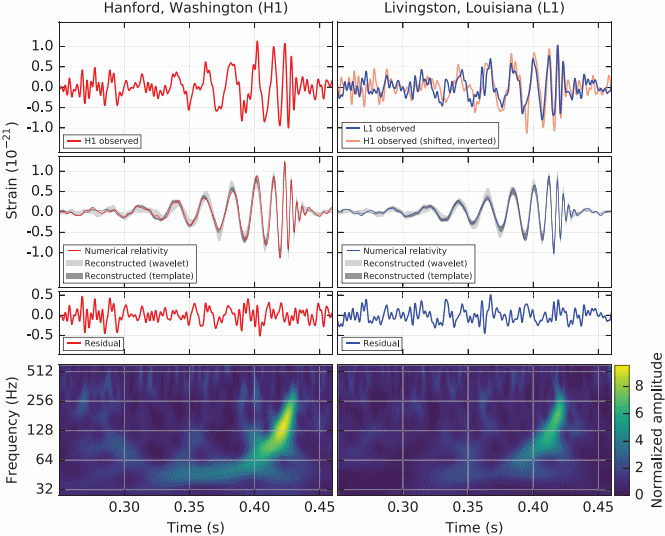The plots depict an event that took place five months ago, on September 14, 2015, when the two observatories of the LIGO experiment simultaneously detected a signal typical of a black hole merger.

The event is attributed to a merger of two black holes, 36 and 29 solar masses in size, respectively, approximately 410 Mpc from the Earth. As the black holes approach each other, their relative velocity approaches the speed of light; after the merger, the resulting object settles down to a rotating Kerr black hole.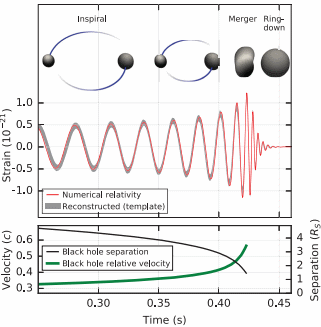When I first heard rumors about this discovery, I was a bit skeptical; black holes of this size (~30 solar masses) have never been observed before. However, I did not realize just how enormous the distance is between us and this event. In such a gigantic volume, it is far less outlandish for such an oddball pair of two very, very massive (but not supermassive!) black holes to exist.

I also didn’t realize just how rapid this event was. I spoke with people previously who were studying the possibility of observing a signal, rising in amplitude and frequency, hours, days, perhaps even weeks before the event. But here, the entire event lasted no more than a quarter of a second. Bang! And something like three solar masses worth of mass-energy are emitted in the form of ripples in spacetime.

The paper is now accepted for publication and every indication is that the group’s work was meticulous. Still, there were some high profile failures recently (OPERA’s faster-than-light neutrinos, BICEP2’s CMB polarization due to gravitational waves) so, as they say, extraordinary claims require extraordinary evidence; let’s see if this detection is followed by more, let’s see what others have to say who reanalyze the data.

But if true, this means that the last great prediction of Einstein is now confirmed through direct observation (indirect observations have been around for about four decades, in the form of the change in the orbital period of close binary pulsars) and also, the last great observational confirmation of the standard model of fundamental physics (the standard model of particle physics plus gravity) is now “in the bag”, so to speak.

All in all, a memorable day.

If you are not following particle physics news or blog sites, you might have missed the big excitement last month when it was announced that the Large Hadron Collider may have observed a new particle with a mass of 750 GeV (roughly 800 times as heavy as a hydrogen atom).

Within hours of the announcement, a flurry of papers began to appear on the manuscript archive, arxiv.org. To date, probably at least 200 papers are there, offering a variety of explanations of this new observation (and incidentally, demonstrating just hungry the theoretical community has become for new data.)

Most of these papers are almost certainly wrong. Indeed, there is a chance that all of them are wrong, on account of the possibility that there is no 750 GeV resonance in the first place.

I am looking at two recent papers. One, by Buckley, discusses what we can (or cannot) learn from the data that have been collected so far. Buckley cautions researchers not to divine more from the data than what it actually reveals. He also remarks on the fact that the observational results of the two main detectors of the LHC, ATLAS and CMS, are somewhat in tension with one another.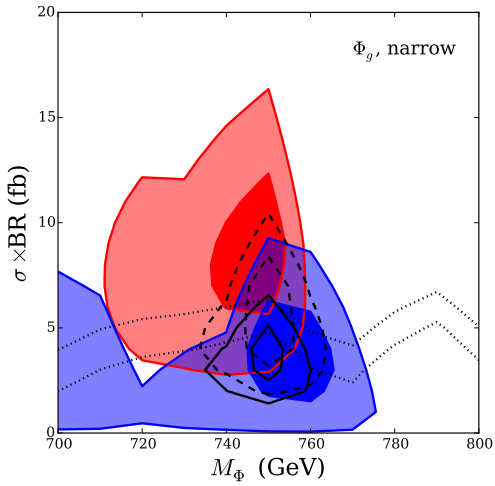From Fig. 2: Best fit regions (1 and 2σ) of a spin-0 mediator decaying to diphotons, as a function of mediator mass and 13 TeV cross section, assuming mediator couplings to gluons and narrow mediator width. Red regions are the 1 and 2σ best-fit regions for the Atlas13 data, blue is the fit to Cms13 data. The combined best fit for both Atlas13 and Cms13 (Combo13) are the regions outlined in black dashed lines. The best-fit signal combination of all four data sets (Combo) is the black solid regions.

The other paper, by Davis et al., is more worrisome. It questions the dependence of the presumed discovery on a crucial part of the analysis: the computation or simulation of background events. The types of reactions that the LHC detects happen all the time when protons collide; a new particle is discerned when it produce some excess events over that background. Therefore, in order to tell if there is indeed a new particle, precise knowledge of the background is of paramount importance. Yet Davis and his coauthors point out that the background used in the LHC data analysis is by no means an unambiguous, unique choice and that when they choose another, seemingly even more reasonable background, the statistical significance of the 750 GeV bump is greatly diminished.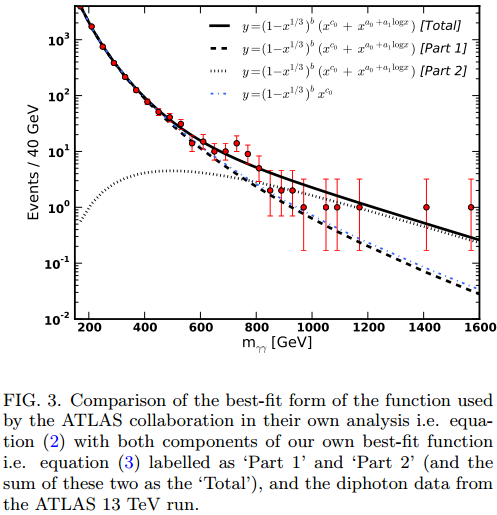I guess we will know more in a few months when the LHC is restarted and more data are collected. It also remains to be seen if the LHC can reproduce the Higgs discovery at its current, 13 TeV operating energy; if it does not, if the Higgs discovery turns out to be a statistical fluke, we may witness one of the biggest embarrassments in the modern history of particle physics.

There is an interesting paper out there by Guerreiro and Monteiro, published a few months ago in Physics Letters A. It is about evaporating black holes. The author’s main assertion is that because of Hawking radiation, not even an infalling ray of light can ever cross the event horizon: rather, the event horizon evaporates faster than the light ray could reach it, neatly solving a bunch of issues and paradoxes associated with black holes and quantum physics, such as the problems with unitarity and information loss.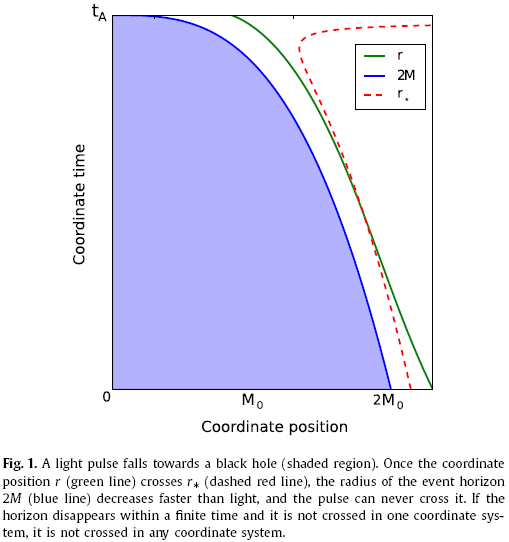I find this idea intriguing and very appealing to my intuition about black holes. I just read the paper and I cannot spot any obvious errors. I am left wondering if the authors appreciated that the Vaydia metric is not a vacuum metric (indeed, it is easy to prove that a spherically symmetric time-dependent solution of Einstein’s field equations cannot be a vacuum solution; there will always be a radial momentum field, carrying matter out of or into the black hole) but it has no bearing on their conclusions I believe.

Now it’s a good question why I am only seeing a paper that is of great interest to me more than six months after its publication. The reason is that although the paper appeared in a pre-eminent journal, it was rejected by the manuscript archive, arxiv.org. This is deeply troubling. The paper is certainly not obviously wrong. It is not plagiarized. Its topic is entirely appropriate to the arXiv subject field to which it was submitted. It is not a duplicate, nor did the authors previously abuse arXiv’s submission system. Yet this paper was rejected. And the most troubling bit is that we do not know why; the rejection policy of arXiv is not only arbitrary, it seems, but also lacks transparency.

This manuscript archive is immensely valuable to researchers. It is one of the greatest inventions of the Internet era. I feel nothing but gratitude towards the people who established and maintain this repository. Nonetheless, I do not believe that such an opaque and seemingly arbitrary rejection policy is justifiable. I hope that this will be remedied and that arXiv’s administrators will take the necessary steps to ensure that in the future, rejections are based on sound criteria and the decisions are transparently explained.

I’ve become a calendar boy.

Or to be more precise, an illustration in a paper that my friend and colleague, Eniko Madarassy and I published together early last year in Physical Review D found its way to the 2016 calendar of the American Physical Society.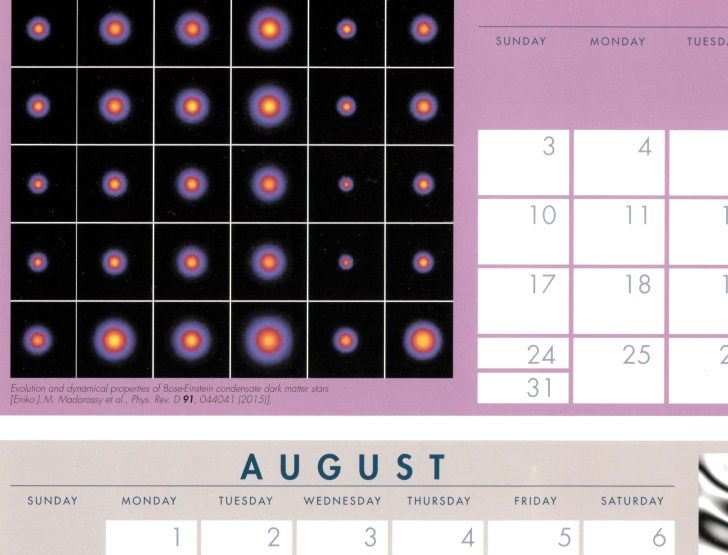Now if only it came with perks, such as getting a discount on my APS membership or something… but no, in fact they didn’t even bother to tell us that this was going to happen, I only found out today when I opened my mailbox and found the calendar inside. Oh well… It was still a nice surprise, so I am not complaining.

It is nice to have a paper accepted on the penultimate day of the year by Physical Review D.

Our paper in question, General relativistic observables for the ACES experiment, is about the Atomic Clock Ensemble in Space (ACES) experiment that will be installed on board the International Space Station (ISS) next year. This experiment places highly accurate atomic clocks in the microgravity environment of the ISS.

How accurate these clocks can be depends, in part, on knowledge of the general relativistic environment in which these clocks will live. This will be determined by the trajectory of the ISS as it travels through the complex gravitational field of the Earth, while being also subject to non-gravitational forces, most notably atmospheric drag and solar radiation pressure.

What complicates the analysis is that the ACES clocks will not be located at the ISS center-of-mass; therefore, as the ISS is quite a large object subject to tidal accelerations, the trajectory of the ACES clocks is non-inertial.

To analyze the problem, we looked at coordinate transformation rules between the various coordinate systems involved: geocentric and terrestrial coordinates, coordinates centered on the ISS center-of-mass, and coordinates centered on ACES.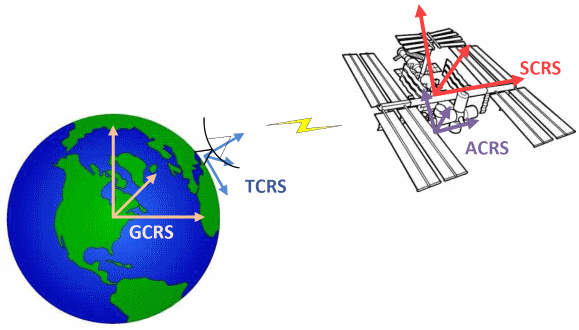One of our main conclusions is that in order for the clock to be fully utilized, the orbit of the ISS must be known at an accuracy of 2 meters or less. This requirement arises if we assume that the orbits are known a priori, and that the clock data are used for science investigations only. If instead, the clock data are used to refine the station orbit, the accuracy requirement is less stringent, but the value of the clock data for scientific analysis is also potentially compromised.

It was an enjoyable paper to work on, and it is nice to end the year on a high note. As we received the acceptance notice earlier today, we were able to put the accepted version on arXiv just in time for it to appear on the very last day of the year, bearing the date December 31, 2015.

Happy New Year!

The reason for my trip to China was to participate in the 3rd workshop on the TianQin mission.

TianQin is a proposed space-borne gravitational wave detector. It is described in our paper, which was recently accepted for publication in Classical and Quantum Gravity. The name, as typical for China, is poetic: it means a zither or harp in space or perhaps (sounds much nicer in English) a celestial harp. A harp that resonates in response to continuous gravitational waves that come from binary pulsars.

Gravitational waves are notoriously hard to detect because they are extremely weak. To date, we only have indirect confirmation of gravitational waves: closely orbiting binary pulsars are known to exhibit orbital decay that is consistent with the predictions of Einstein’s gravity.

Gravitational radiation is quadrupole radiation. It means basically that it simultaneously squeezes spacetime in one direction and stretches it in a perpendicular direction. This leads to the preferred method of detection: two perpendicular laser beams set to interfere with each other. As a gravitational wave passes through, a phase shift occurs as one beam travels a slightly longer, the other a slightly shorter distance. This phase shift manifests itself as an interference pattern, which can be detected.

But detection is much harder in practice than it sounds. Gravitational waves are not only very weak, they are also typically very low in frequency. Strong gravitational waves (relatively speaking) are produced by binaries such as HM Cancri (aka. RX J0806.3+1527) but even such an extreme binary system has an orbital period of several minutes. The corresponding gravitational wave frequency is measured in millihertz, and the wavelength, in tens or hundreds of millions of kilometers.

There is one exception: inspiraling neutron star or black hole binary systems at the very end of their lives. These could produce detectable gravitational waves with frequencies up to even a kilohertz or so, but these are random, transient events. Nonetheless, there are terrestrial detectors such as LIGO (Laser Interferometer Gravitational-wave Observatory) that are designed to detect such events, and the rumor I heard is that it may have already happened. Or not… let’s wait for the announcement.

But the continuous waves from close binaries require a detector comparable in size to the wavelength of their gravitational radiation. In short, an interferometer in which the laser beams can travel at least a few hundred thousand kilometers, preferably more. Which means that the interferometer must be in space.

This is the idea behind LISA, the Laser Interferometer Space Antenna project. Its current incarnation is eLISA (the “e” stands for “evolved”), a proposed European Space Agency mission, a precursor of which, LISA Pathfinder, was launched just a few days ago. Nonetheless, eLISA’s future remains uncertain.

Enter the Chinese, with TianQin. Whereas eLISA’s configuration of three spacecraft is designed to be in deep space orbiting one of the Earth-Sun Lagrange points with inteferometer arm lengths as long as 1.5 million kilometers, TianQin’s more modest proposal calls for a geocentric configuration, with arm lengths of 150,000 km or so. This means reduced sensitivity, of course, and the geocentric orbit introduces unique challenges. Nonetheless, our colleagues believe that it is fundamentally feasible for TianQin to detect gravitational waves from a known source with sufficient certainty. In other words, the primary mission objective of TianQin is to serve as a gravitational wave detector, confirming the existence of continuous waves emitted by a known binary system, as opposed to being an observatory, usable to find previously unknown sources of gravitational radiation. Detection is always easier: in radio technology, for instance, a lock-in amplifier can be used to detect the presence of a carrier wave even when it is far too weak to carry any useful information.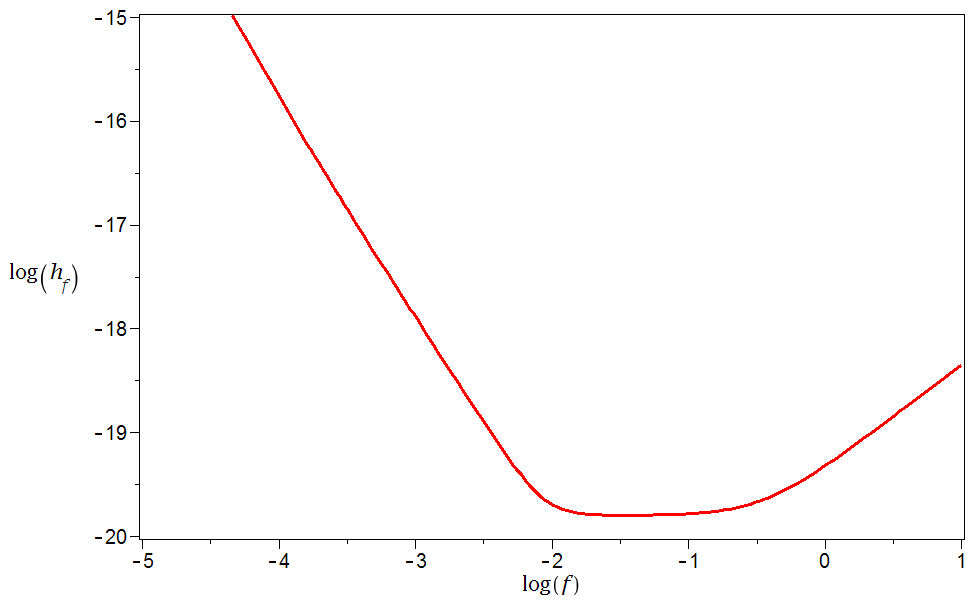Theoretical sensitivity curve of the proposed TianQin mission.

The challenges of TianQin are numerous, but here are a few main ones:

• First, precisely controlling the orbits of shielded, drag-free test masses such that their acceleration due to nongravitational forces is less than $$10^{-15}~{\rm m}/{\rm s}^2$$.
• Second, precisely controlling the optical path such that no unmodeled effects (e.g., thermal expansion due to solar heating) contribute unmodeled changes more than a picometer in length.
• Third, implementing time-delay interferometry (TDI), which is necessary in order to be able to compare the phases of laser signals that traveled different lengths, and do so with sufficient timing accuracy to minimize the contributions due to fluctuations in laser frequency.

Indeed, some of the accuracy requirements of TianQin exceed those of eLISA. This is a tall order for any space organization, and China is no exception. Still, as they say, where there is a will…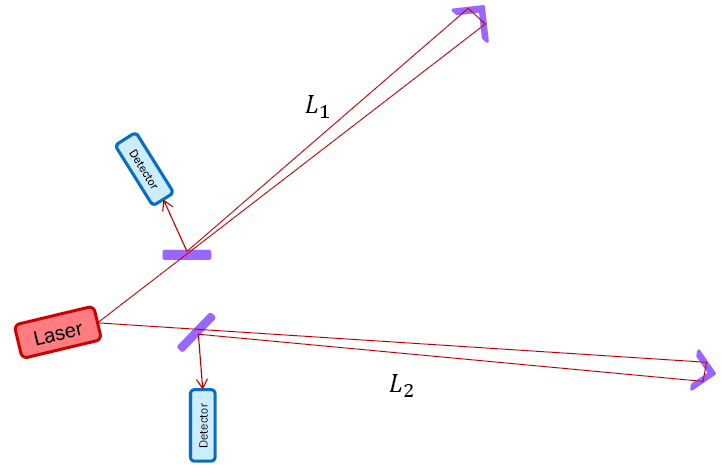Unequal-arm Michelson interferometer.

One thing that complicates matters is that there are legal barriers when it comes to cooperation with China. In the United States there are strong legal restrictions preventing NASA and researchers at NASA from cooperating with Chinese citizens and Chinese enterprises. (Thankfully, Canada is a little more open-minded in this regard.) Then there is the export control regime: Technologies that can be utilized to navigate ballistic missiles, to offer satellite-based navigation on the ground, and to perform remote sensing may be categorized as munitions and fall under export control restrictions in North America, with China specifically listed as a proscribed country.

The know-how (and software) that would be used to navigate the TianQin constellation is arguably subject to such restrictions at least on the first two counts, but possibly even the third: a precision interferometer in orbit can be used for gravitiational remote sensing, as it has been amply demonstrated by GRACE (Gravity Recovery And Climate Experiment), which was orbiting the Earth, and GRAIL (Gravity Recovery And Interior Laboratory) in lunar orbit. Then there is the Chinese side of things: precision navigation requires detailed information about the capabilities of tracking stations in China, which may be, for all I know, state secrets.

While these issues make things a little tricky for Western researchers, TianQin nonetheless has a chance of becoming a milestone experiment. I sincerely hope that they succeed. And I certainly feel honored, having been invited to take part in this workshop.

Hello, Guangzhou. And hello world, from Guangzhou. Here is what I see from my hotel window today:It is a very interesting place. Today, I had a bit of a walk not just along the main urban avenues, full of neon and LED signs and modern high-tech stores, but also in some of the back alleys, complete with street vendors, stray dogs, and 60-70 year old crumbling buildings, some abandoned. In short… a real city with a real history.

For what it’s worth, I am here on account of a conference about a planned space-borne gravitational wave detector called TianQin.

It’s time for me to write about physics again. I have a splendid reason: one of the recipients of this year’s physics Nobel is from Kingston, Ontario, which is practically in Ottawa’s backyard. He is recognized for his contribution to the discovery of neutrino oscillations. So I thought I’d write about neutrino oscillations a little.

Without getting into too much detail, the standard way of describing a theory of quantum fields is by writing down the so-called Lagrangian density of the theory. This Lagrangian density represents the kinetic and potential energies of the system, including so-called “mass terms” for fields that are massive. (Which, in quantum field theory, is the same as saying that the particles we associate with the unit oscillations of these fields have a specific mass.)

Now most massive particles in the Standard Model acquire their masses by interacting with the celebrated Higgs field in various ways. Not neutrinos though; indeed, until the mid 1990s or so, neutrinos were believed to be massless.

But then, neutrino oscillations were discovered and the physics community began to accept that neutrinos may be massive after all.

So what is this about oscillations? Neutrinos are somewhat complicated things, but I can demonstrate the concept using two hypothetical “scalar” particles (doesn’t matter what they are; the point is, their math is simpler than that of neutrinos.) So let’s have a scalar particle named $$\phi$$. Let’s suppose it has a mass, $$\mu$$. The mass term in the Lagrangian would actually be in the form, $$\frac{1}{2}\mu\phi^2$$.

Now let’s have another scalar particle, $$\psi$$, with mass $$\rho$$. This means another mass term in the Lagrangian: $$\frac{1}{2}\rho\psi^2$$.

But now I want to be clever and combine these two particles into a two-element abstract vector, a “doublet”. Then, using the laws of matrix multiplication, I could write the mass term as

$$\frac{1}{2}\begin{pmatrix}\phi&\psi\end{pmatrix}\cdot\begin{pmatrix}\mu&0\\0&\rho\end{pmatrix}\cdot\begin{pmatrix}\phi\\\psi\end{pmatrix}=\frac{1}{2}\mu\phi^2+\frac{1}{2}\rho\psi^2.$$

Clever, huh?

But now… let us suppose that there is also an interaction between the two fields. In the Lagrangian, this interaction would be represented by a term such as $$\epsilon\phi\psi$$. Putting $$\epsilon$$ into the “0” slots of the matrix, we get

$$\frac{1}{2}\begin{pmatrix}\phi&\psi\end{pmatrix}\cdot\begin{pmatrix}\mu&\epsilon\\\epsilon&\rho\end{pmatrix}\cdot\begin{pmatrix}\phi\\\psi\end{pmatrix}=\frac{1}{2}\mu\phi^2+\frac{1}{2}\rho\psi^2+\epsilon\phi\psi.$$

And here is where things get really interesting. That is because we can re-express this new matrix using a combination of a diagonal matrix and a rotation matrix (and its transpose):

$$\begin{pmatrix}\mu&\epsilon\\\epsilon&\rho\end{pmatrix}=\begin{pmatrix}\cos\theta/2&\sin\theta/2\\-\sin\theta/2&\cos\theta/2\end{pmatrix}\cdot\begin{pmatrix}\hat\mu&0\\0&\hat\rho\end{pmatrix}\cdot\begin{pmatrix}\cos\theta/2&-\sin\theta/2\\\sin\theta/2&\cos\theta/2\end{pmatrix},$$

which is equivalent to

$$\begin{pmatrix}\hat\mu&0\\0&\hat\rho\end{pmatrix}=\begin{pmatrix}\cos\theta/2&-\sin\theta/2\\\sin\theta/2&\cos\theta/2\end{pmatrix}\cdot\begin{pmatrix}\mu&\epsilon\\\epsilon&\rho\end{pmatrix}\cdot\begin{pmatrix}\cos\theta/2&\sin\theta/2\\-\sin\theta/2&\cos\theta/2\end{pmatrix},$$

or

\begin{pmatrix}\hat\mu&0\\0&\hat\rho\end{pmatrix}=\frac{1}{2}\begin{pmatrix}\mu+\rho+(\mu-\rho)\cos\theta-2\epsilon\sin\theta&(\rho-\mu)\sin\theta-2\epsilon\cos\theta\$$\rho-\mu)\sin\theta-2\epsilon\cos\theta&\mu+\rho+(\rho-\mu)\cos\theta+2\epsilon\sin\theta\end{pmatrix}, which tells us that \(\tan\theta=2\epsilon/(\rho-\mu)$$, which works so long as $$\rho\ne\mu$$. Now why is this interesting? Because we can now write \begin{align}\frac{1}{2}&\begin{pmatrix}\phi&\psi\end{pmatrix}\cdot\begin{pmatrix}\mu&\epsilon\\\epsilon&\rho\end{pmatrix}\cdot\begin{pmatrix}\phi\\\psi\end{pmatrix}\\ &{}=\frac{1}{2}\begin{pmatrix}\phi&\psi\end{pmatrix}\cdot\begin{pmatrix}\cos\theta/2&\sin\theta/2\\-\sin\theta/2&\cos\theta/2\end{pmatrix}\cdot\begin{pmatrix}\hat\mu&0\\0&\hat\rho\end{pmatrix}\cdot\begin{pmatrix}\cos\theta/2&-\sin\theta/2\\\sin\theta/2&\cos\theta/2\end{pmatrix}\cdot\begin{pmatrix}\phi\\\psi\end{pmatrix}\\ &{}=\frac{1}{2}\begin{pmatrix}\hat\phi&\hat\psi\end{pmatrix}\cdot\begin{pmatrix}\hat\mu&0\\0&\hat\rho\end{pmatrix}\cdot\begin{pmatrix}\hat\phi\\\hat\psi\end{pmatrix}.\end{align} What just happened, you ask? Well, we just rotated the abstract vector $$(\phi,\psi)$$ by the angle $$\theta/2$$, and as a result, diagonalized the expression. Which is to say that whereas previously, we had two interacting fields $$\phi$$ and $$\psi$$ with masses $$\mu$$ and $$\rho$$, we now re-expressed the same physics using the two non-interacting fields $$\hat\phi$$ and $$\hat\psi$$ with masses $$\hat\mu$$ and $$\hat\rho$$. So what is actually taking place here? Suppose that the doublet $$(\phi,\psi)$$ interacts with some other field, allowing us to measure the flavor of an excitation (particle) as being either a $$\phi$$ or a $$\psi$$. So far, so good. However, when we attempt to measure the mass of the doublet, we will not measure $$\mu$$ or $$\rho$$, because the two states interact. Instead, we will measure $$\hat\mu$$ or $$\hat\rho$$, corresponding to the states $$\hat\phi$$ or $$\hat\psi$$, respectively: that is, one of the mass eigenstates. Which means that if we first perform a flavor measurement, forcing the particle to be in either the $$\phi$$ or the $$\psi$$ state, followed by a mass measurement, there will be a nonzero probability of finding it in either the $$\hat\phi$$ or the $$\hat\psi$$ state, with corresponding masses $$\hat\mu$$ or $$\hat\rho$$. Conversely, if we first perform a mass measurement, the particle will be either in the $$\hat\phi$$ or the $$\hat\psi$$ state; a subsequent flavor measurement, therefore, may give either $$\phi$$ or $$\psi$$ with some probability. In short, the flavor and mass eigenstates do not coincide. This is more or less how neutrino oscillations work (again, omitting a lot of important details), except things get a bit more complicated, as neutrinos are fermions, not scalars, and the number of flavors is three, not two. But the basic principle remains the same. This is a unique feature of neutrinos, by the way. Other particles, e.g., charged leptons, do not have mass eigenstates that are distinct from their flavor eigenstates. The mechanism that gives them masses is also different: instead of a self-interaction in the form of a mass matrix, charged leptons (as well as quarks) obtain their masses by interacting with the Higgs field. But that is a story for another day. I woke up this morning to the news that Mexican-Israeli physicist Jacob Bekenstein died two days ago, at the age of 68, in Helsinki, Finland. I saw nothing about the cause of death.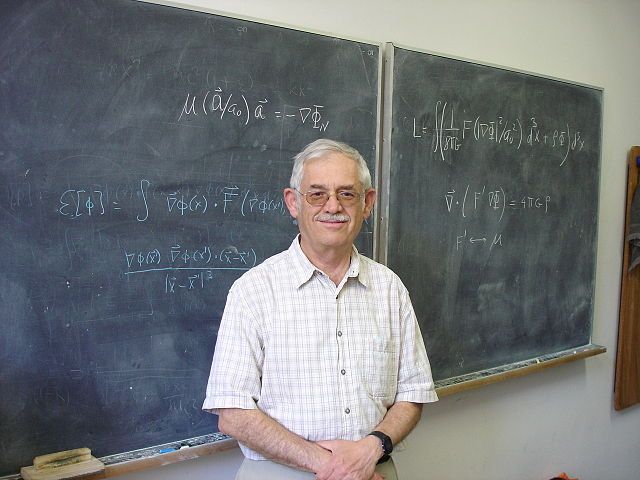Bekenstein’s work is well known to folks dealing with gravity theory. Two of his contributions stand out in particular. First, Bekenstein was first to suggest that black holes should have entropy. His work, along with that of Stephen Hawking, led to the Bekenstein-Hawking entropy formula $$S=kc^3A/4G\hbar$$, relating the black hole’s surface area $$A$$ to its entropy $$S$$ using the speed of light $$c$$, the gravitational constant $$G$$, the reduced Planck constant $$\hbar$$ and Boltzmann’s constant $$k$$. With this work, the science of black hole thermodynamics was born, leading to all kinds of questions about the nature of black holes and the connection between thermodynamics and gravity, many of which remain unanswered to this day. Bekenstein’s second contribution was to turn Morehai Milgrom’s MOdified Newtonian Dynamics (MOND) into a respectable relativitistic theory. The MOND paradigm is about replacing Newton’s law relating force $$({\mathbf F})$$, mass $$(m)$$ and acceleration $$({\mathbf a})$$, $${\mathbf F}=m{\mathbf a}$$, with the modified law $${\mathbf F}=\mu(a/a_0)m{\mathbf a}$$, where all we know about the function $$\mu(x)$$ is that $$\lim_{x\to 0}\mu(x)=x$$ and $$\lim_{x\to\infty}\mu(x)=1$$. Surprisingly, the right choice of $$a_0$$ results in an acceleration law that explains the anomalous rotation of galaxies without the need for dark matter. However, in this form, MOND is theoretically ugly: it is a formula that violates basic conservation laws, including the consevation of energy, for instance. Bekenstein’s TeVeS (Tensor-Vector-Scalar) gravity theory provides a general relativistic framework for MOND, one that does respect basic conservation laws, yet reproduces the MOND acceleration formula in the low energy limit. I never met Jacob Bekenstein, and now I never will. A pity. May he rest in peace. Here is one of the most mind-boggling animation sequences that I have ever seen: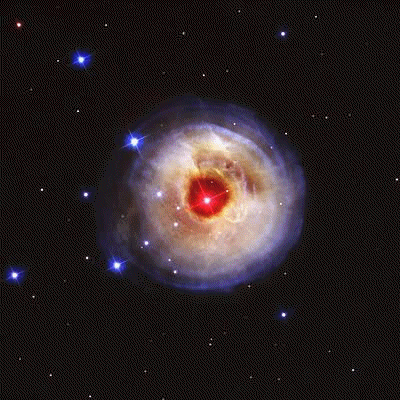This image depicts V838 Monocerotis, a red variable star that underwent a major outburst back in 2002. Why do I consider this animation mind-boggling? Because despite all appearances, it is not an expanding shell of dust or gas. Rather, it is echoes of the flash of light, reflected by dust situated behind the star, reaching our eyes several years after the original explosion. In other words, this image represents direct, visual evidence of the finite speed of light. The only comparable thing that I can think of is this video, created a few years ago using tricky picosecond photography, of a laser pulse traveling in a bottle. However, unlike that video, the images of V838 Monocerotis required no trickery, only a telescope. And light echoes are more than mere curiosities: they actually make it possible to study past events. Most notably, a faint light echo of a supernova that was observed nearly half a millennium ago, in 1572, was detected in 2008. $$\renewcommand{\vec}{\boldsymbol{\mathrm{#1}}}$$Continuing something I began about a month ago, I spent more of my free time than I care to admit re-deriving some of the most basic identities in quantum physics.I started with the single-particle case of a harmonic oscillator. Such an oscillator is characterized by the classical LagrangianL=\frac{1}{2}m\dot{\vec{q}}^2-\frac{1}{2}k\vec{q}^2-V(\vec{q}),$$and the corresponding Hamiltonian$$H=\frac{\vec{p}^2}{2m}+\frac{1}{2}k\vec{q}^2+V(\vec{q}).$$By multiplying this Hamiltonian with $$\psi=e^{i(\vec{p}\cdot\vec{q}-Ht)/\hbar}$$, we basically obtain Schrödinger’s equation:$$\left[i\hbar\partial_t+\frac{\hbar^2}{2m}\vec{\nabla}^2-\frac{1}{2}k\vec{q}^2-V(\vec{q})\right]e^{i(\vec{p}\cdot\vec{q}-Ht)/\hbar}=0.$$The transition to the quantum theory begins when we accept that linear combinations of solutions of this equation (i.e., $$\psi$$-s corresponding to different values of $$\vec{p}$$ and $$H$$) also represent physical states of the system, despite the fact that these “mixed” solutions are not eigenfunctions and there are no corresponding classical eigenvalues $$\vec{p}$$ and $$H$$. Pure algebra can lead to an expression of $$\hat{H}$$ in the form of “creation” and “annihilation” operators:$$\hat{H}=\hbar\omega\left(\hat{a}^\dagger\hat{a}+\frac{1}{2}\right)+V(\vec{q}).These operators have the properties \begin{align*} \hat{H}\hat{a}\psi_n&=\left([\hat{H},\hat{a}]+\hat{a}\hat{H}\right)\psi_n=(E_n-\hbar\omega)\hat{a}\psi_n,\\ \hat{H}\hat{a}^\dagger\psi_n&=\left([\hat{H},\hat{a}^\dagger]+\hat{a}^\dagger\hat{H}\right)\psi_n=(E_n+\hbar\omega)\hat{a}^\dagger\psi_n. \end{align*} whereE_n=\left(n+\frac{1}{2}\right)\hbar\omega.$$This same derivation can be done in the relativistic single particle case as well. Moreover, it is possible to define a classical scalar field in the form$${\cal L}=\frac{1}{2}\rho(\partial_t\phi)^2-\frac{1}{2}\rho c^2(\vec{\nabla}\phi)^2-\frac{1}{2}\kappa\phi^2-V(\phi),$$which leads to the Hamiltonian density$${\cal H}=\pi\partial_t\phi-{\cal L}=\frac{\pi^2}{2\rho}+\frac{1}{2}\rho c^2(\vec{\nabla}\phi)^2+\frac{1}{2}\kappa\phi^2+V(\phi).$$The transitioning to the quantum theory occurs by first expressing $$\phi$$ as a Fourier integral and then promoting the Fourier coefficients to operators that satisfy a commutation relation in the form$$[\hat{a}(\omega,\vec{k}),\hat{a}^\dagger(\omega,\vec{k}’)]=(2\pi)^3\delta^3(\vec{k}-\vec{k}’).$$This leads to a commutation relation for the field and its canonical momentum in the form$$[\hat{\phi}(t,\vec{x}),\hat{\pi}(t,\vec{x}’)]=i\hbar\delta^3(\vec{x}-\vec{x}’),$$and for the Hamiltonian,$$\hat{H}=\hbar\omega\left\{\frac{1}{2}+\int\frac{d^3\vec{k}}{(2\pi)^3}\hat{a}^\dagger(\omega,\vec{k})\hat{a}(\omega,\vec{k})\right\}+\int d^3xV(\hat{\phi}).$$More details are provided on my Web site, at https://www.vttoth.com/CMS/physics-notes/297. So why did I find it necessary to capture here something that can be found in first chapter of every semi-decent quantum field theory textbook? Several reasons. • First, I wanted to present a consistent treatment of all four cases: the nonrelativistic and relativistic case for both the particle and the field theory. • Second, I wanted to write down all relevant equations without omitting dimensions. I wanted to write down a Lagrangian density that has the dimensions of energy density, consistent with a scalar field that has the dimensions of length (i.e., a displacement). • Third, I wanted to spell out some of the details of the derivation that are omitted from nearly all textbooks yet, I am obliged to admit, almost stumped me. That is, once you see the derivation the steps are reasonably trivial, but it is still hard to stumble upon exactly the right way to apply the relevant identities related to Fourier transforms and Dirac deltas. • Lastly, I find it revealing how this approach can highlight exactly where a quantum theory is introduced. In the particle theory case, it is when we assume that “mixed states”, that is, linear combinations of eigenstates also represent physical states of a system, despite the fact that they do not correspond to classical eigenvalues. In the case of a field theory, the transition occurs when we replace Fourier coefficients with operators: implicit in the transition is that once again, mixed states are included as representing actual physical states of the system. Note also how none of this has anything to do with interpretations. There is no “collapse of the wave function” or any such nonsense. That stuff happens when we introduce into our consideration a “measurement event”, effectively an interaction between the quantum system and a classical instrument, which forces the quantum system into an eigenstate. This eigenstate cannot be predicted from the initial conditions alone, precisely because the classical idealization of the measurement apparatus effectively amounts to an admission of ignorance about its true quantum state. There is a particularly neat way to derive Schrödinger’s equation, and to justify the “canonical substitution” rules for replacing energy and momentum with corresponding operators when we “quantize” an equation. Take a particle in a potential. Its energy is given by$$E=\frac{{\bf p}^2}{2m}+V({\bf x}),$$or$$E-\frac{{\bf p}^2}{2m}-V({\bf x})=0.$$Now multiply both sides this equation by the formula $$e^{i({\bf p}\cdot{\bf x}-Et)/\hbar}$$. We note that this exponential expression cannot ever be zero if the part in the exponent that’s in parentheses is real:$$\left[E-\frac{{\bf p}^2}{2m}-V({\bf x})\right]e^{i({\bf p}\cdot{\bf x}-Et)/\hbar}=0.$$So far so good. But now note that$$Ee^{i({\bf p}\cdot{\bf x}-Et)/\hbar}=i\hbar\frac{\partial}{\partial t}e^{i({\bf p}\cdot{\bf x}-Et)/\hbar},$$and similarly,$${\bf p}^2e^{i({\bf p}\cdot{\bf x}-Et)/\hbar}=-\hbar^2{\boldsymbol\nabla}e^{i({\bf p}\cdot{\bf x}-Et)/\hbar}.$$This allows us to rewrite the previous equation as$$\left[i\hbar\frac{\partial}{\partial t}+\hbar^2\frac{{\boldsymbol\nabla}^2}{2m}-V({\bf x})\right]e^{i({\bf p}\cdot{\bf x}-Et)/\hbar}=0.$$Or, writing $$\Psi=e^{i({\bf p}\cdot{\bf x}-Et)/\hbar}$$ and rearranging:$$i\hbar\frac{\partial}{\partial t}\Psi=-\hbar^2\frac{{\boldsymbol\nabla}^2}{2m}\Psi+V({\bf x})\Psi,$$which is the good old Schrödinger equation. The method works for an arbitrary, generic Hamiltonian, too. Given$$H({\bf p})=E,$$we can write$$\left[E-H({\bf p})\right]e^{i({\bf p}\cdot{\bf x}-Et)/\hbar}=0,$$which is equivalent to$$\left[i\hbar\frac{\partial}{\partial t}-H(-i\hbar{\boldsymbol\nabla})\right]\Psi=0.

So if this equation is identically satisfied for a classical system with Hamiltonian $$H$$, what’s the big deal about quantum mechanics? Well… a classical system satisfies $$E-H({\bf p})=0$$, where $$E$$ and $${\bf p}$$ are eigenvalues of the differential operators $$i\hbar\partial/\partial t$$ and $$-i\hbar{\boldsymbol\nabla}$$, respectively. Schrödinger’s equation, on the other hand, remains valid in the general case, not just for the eigenvalues.